# Decimals

Here is what Macdonald has written in his notebook about decimals:

Decimals are fractions having for their denominators the powers of the number 10, that is, unity with cyphers annexed; thus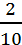,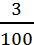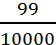are decimal fractions. In the notation of decimals the denominators are not written; but they are indicated by prefixing a point (∙) before the numerators and in any given decimal, the denominator, in general, consists of unity with as many cyphers annexed to it as there are figures after the point; the decimal fraction,,are written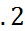,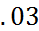,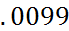.

Decimals consisting of the same number of figures, have therefore the same denominator and in decimals consisting of different numbers of figures, those figures at equal distances from the point will have the same denominators;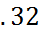have the same denominator, viz. (100) and in the decimals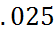&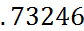the 5 in the one and the 2 in the other have the same denominator (viz. 1000).

If the decimal consist of 1 figure, its denominator is 10; if of 2, 100; if of 3, 1000 &c – Hence the first figure of a decimal may be called tenths; the second hundredths; the third thousandths; &c; thus, we read the decimal,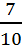,,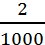,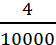, or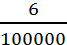hundredths of thousandths.

The number Unity is greatest than the greatest possible decimal number, greater, for instance, than .999995 – hence, in numbers consisting partly of integers and partly of decimals, that which has the greatest integer, is the greater; thus 5.02 is greater than 4.998.

Note. As in notation of integers, the values of the figures increase in a tenfold proportion from the right hand to the left, so, in decimals, or their values, decrease in a tenfold proportion from the left hand to the right. The operations, in the arithmetic of decimals, are all performed in the same manner as the corresponding operations in the arithmetic of integers, only care must be taken in distinguishing the decimal part of every result.